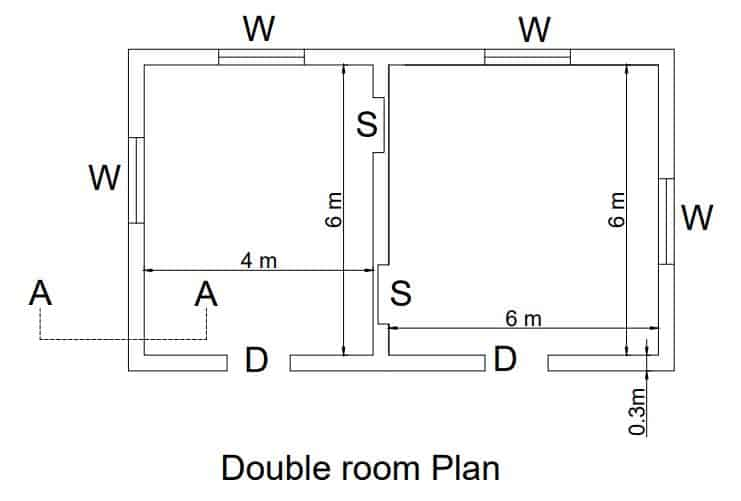# Double room building estimation|6 stepsDouble room building estimation can be calculated by estimating earthwork in excavation,foundation concrete,plinth beam,1st class brickwork, cement concrete quantity.

## Double room building estimation specifications, drawings

The following are the double room building estimation specifications

• In foundation cement concrete M25 is utilised.
• CRS wall is utilised in the foundation for the base of the brick wall.
• Plinth beam 400 mm x 600mm is utilised in cement concrete M25
• Dampproof course 25mm thick is applied.
• In superstructure 1st class brickwork in 1:4 cement mortar and thickness 300mm is utilised.
• Doors D ,size-1.2 m x 2.1 m
• windows W,size-1 m x 1.5 m,Shelves S-1 m x 1.5 m

## Double room building estimation in 6 steps

The following building estimation 7 steps for a double room.

1. In foundation excavated earth quantity,
2. In foundation cement concrete quantity,
3. CRS wall in the foundation quantity,
4. Plinth beam quantity
5. Dampproof course quantity,
6. In superstructure 1st class brickwork quantity

Note: This building estimation is only limited up to brick walls quantity in the superstructure.

Length of the long wall from centre to centre =4 m + 6 m + 0.3 m+0.5×0.3 m +0.5×0.3 m=10.6 m

length of short walls from centre to centre = 6 m +0.5×0.3 m +0.5×0.3 m=6.3 m

### 1.Excavated earth quantity in foundation

Longwall length of excavated earth in foundation can be calculated by =10.6 m +0.5 x 1.1 m +0.5×1.1 m=11.7 m

Short wall length of excavated earth in foundation can be calculated by =6.3 m -0.5×1.1 m-0.5×1.1 m=5.2 m

### 2.Cement concrete quantity in foundation

Longwall length of cement concrete earth in foundation can be calculated by =10.6 m +0.5 x 1.1 m +0.5×1.1 m=11.7 m

Short wall length of cement concrete in foundation can be calculated by =6.3 m -0.5×1.1 m-0.5×1.1 m=5.2 m

### 3.CRS wall quantity in foundation

#### Long wall length of CRS wall in foundation

In 1st footing can be calculated by =10.6 m +0.5 x 0.8 m +0.5 x 0.8 m=11.4 m

In 2nd footing can be calculated by =10.6 m +0.5 x 0.7 m +0.5 x 0.7 m=11.3 m

In 3rd footing can be calculated by =10.6 m +0.5 x 0.6 m +0.5 x 0.6 m=11.2 m

In 4th footing can be calculated by =10.6 m +0.5 x 0.5 m +0.5 x 0.5 m=11.1 m

#### Short wall length of CRS wall in foundation

In 1st footing can be calculated by =6.3 m -0.5 x 0.8 m -0.5 x 0.8 m=5.5 m

In 2nd footing can be calculated by =6.3 m -0.5 x 0.7 m -0.5 x 0.7 m=5.6 m

In 3rd footing can be calculated by =6.3 m -0.5 x 0.6 m -0.5 x 0.6 m=5.7 m

In 4th footing can be calculated by =6.3 m -0.5 x 0.5 m -0.5 x 0.5 m=5.8 m

### 4.Dampproof course quantity

25 mm thick damp proof course is laid and considered in building estimation.

Longwall length for damp proof course can be calculated by =10.6 m +0.4 x 0.5 m +0.4×0.5 m=11 m

Short wall length for damp proof course can be calculated by =6.3 m -0.5×0.4 m-0.5×0.4 m=5.9 m

### 5.Plinth beam quantity

Longwall length of plinth beam can be calculated by =10.6 m +0.4 x 0.5 m +0.4 x 0.5 m=11 m

Short wall length of plinth beam can be calculated by =6.3 m -0.5 x 0.4 m-0.5 x 0.4 m=5.9 m

### 6.1st class brick work quantity in superstructure

1st class brick work is laid in 1:4 cement mortar with 300 mm thick in building estimation is used.

Center to center Long-wall length of brick wall can be calculated by =10.6 m +0.3 x 0.5 m +0.3 x 0.5 m=10.9 m

Center to center Short wall length of plinth beam can be calculated by =6.3 m -0.3 x 0.4 m-0.3 x 0.4 m=6 m

### Conclusion

So from the above 6 steps double room building estimation up to brick wall in superstructure can be done easily.